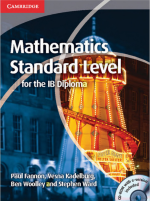To provide the best private education possible for our students we are looking for experienced teachers.
Vacancies are available throughout the year. To join our team please first fill in this form, then, email your application letter
enclosing a detailed CV to recruit@ahmath.com.
If you have any inquiries, please e-mail info@ahmath.com.

E-mail: info@ahmath.com

AhMath Real than i, rational than π

# IB (International Baccalaureate)

Prep by AhMath's HQ Teachers. Get what you need to succeed! This class will introduce you to the skills and strategies necessary for studying for the IB (International Baccalaureate) mock exams. We offer
• Taught by Mathematics specialist teachers,
• Calculator Training Session,
• Past Papers,
• Strategies for Tackling the Different Question Types on the IB Mock exams,
• Skill Review in Math
• Targeted online and printed Homework,
• Suggestions for Further Self-Study.
The International Baccalaureate (IB), formerly known as the International Baccalaureate Organization (IBO), is an international educational foundation headquartered in Geneva, Switzerland and founded in 1968. It offers three educational programmes: the IB Diploma Programme and the IB Career-related Programme for students aged 16 to 19, the IB Middle Years Programme (MYP), designed for students aged 11 to 16, and the IB Primary Years Programme (PYP) for children aged 3 to 12. To teach these programmes, schools need to be authorised by IB.

You need to log in to reach the questions.

### Best IB Instructors

#### Ahmet Arduc (Math)Distance is not a matter: AhMath uses the best online collaboration system. What you need is just an internet-connected computer and headphone with microphone. No need to download anything. This way you can get help anytime, anywhere.Get textbook solutions and 24/7 study help for your courses with AhMath

If you need any help, do not hesitate to contact AhMath and just let me know.

##### 7-page solution booklet for the VECTOR APPLICATIONS (Chapter 13)## IB Math SL Revision Course

Online

HKD600/hr

Quadratic functions Exponents and logarithms Algebraic structures The theory of functions
Transformations of graphs Sequences and series Binomial expansion Circular measure and trigonometric functions Trigonometric equations and identities
Geometry of triangles and circles
Vectors Basic differentiation and its applications
Basic integration and its applications Further differentiation Further integration Summarising data Probability Probability distributions
Questions crossing chapters

## IB Math SL Revision Course

Face-to-Face

HKD750/hr

Quadratic functions Exponents and logarithms Algebraic structures The theory of functions
Transformations of graphs Sequences and series Binomial expansion Circular measure and trigonometric functions Trigonometric equations and identities
Geometry of triangles and circles
Vectors Basic differentiation and its applications
Basic integration and its applications Further differentiation Further integration Summarising data Probability Probability distributions
Questions crossing chapters

## IB Math HL Revision Course

Online

HKD650/hr

Trigonometry I Quadratic Equations, Functions and Inequalities Functions
Polynomials Exponentials and Logarithmic Functions
Sequences, Series and Binomial Theorem Trigonometry II
Differentiation I (Introduction)
Differentiation II (Further Techniques) Differentiation III (Applications) Vectors Techniques Vectors, Lines and Planes
Integration I Integration II (Further Techniques) Integration II (Applications) Complex Numbers Mathematical Induction
Statistics Probability
Discrete Probability Distributions
Continues Probability Distributions

## IB Math HL Revision Course

Face-to-Face

HKD800/hr

Trigonometry I Quadratic Equations, Functions and Inequalities Functions
Polynomials Exponentials and Logarithmic Functions
Sequences, Series and Binomial Theorem Trigonometry II
Differentiation I (Introduction)
Differentiation II (Further Techniques) Differentiation III (Applications) Vectors Techniques Vectors, Lines and Planes
Integration I Integration II (Further Techniques) Integration II (Applications) Complex Numbers Mathematical Induction
Statistics Probability
Discrete Probability Distributions
Continues Probability Distributions

Go to top of page

# IB Classes Enrolment Form

Please complete the following application if you are seeking a HQT.